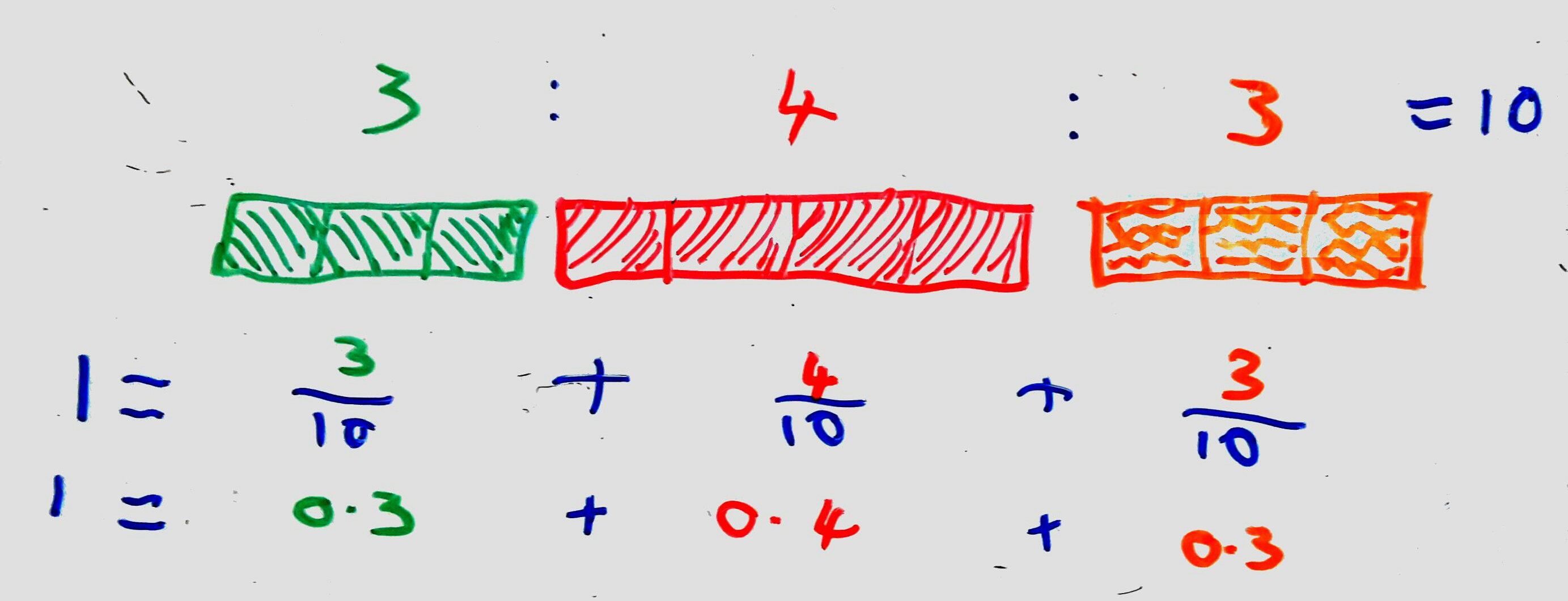### Let's build a weighted random number generator!

Ever wondered how random loot in a dungeon is generated? Or how the rooms in a procedurally generated castle might be picked? Perhaps you need to skew the number of times an apple is picked by your game engine over a banana. If you've considered any of these things, then you want a weighted random number generator. In this post, I'll be showing you how I built one, and how you can build one too.

If you're interested in trying to build one for yourself first though, then look away now! Come back when you're done (or stuck) to see my solution.

To start with, let's consider what a weighted random number generator actually is. Let's say we've got 3 rewards for a treasure chest: a cool-looking shield, a health potion, and a fancy ring. We want to give the player 1 of the 3 when they option the chest, making sure that the health potion is more common than the others. We can represent that as a ratio: $3 : 4 : 3$.(Above: The ratio between the different items. See below for the explanation of the math!).

In order to pick one of the 3 items using the ratio, we need to normalise the ratio so that it's between $0$ and $1$. That's rather easy, as far as maths goes: All we have to do is convert each part of the ratio into a fraction, and that into a decimal. Let's calculate the denominator of the fraction first. That's easy-peasy too - we just add up all the parts of the ratio, as we want to represent each part as a fraction of a whole: $3 + 4 + 3 = 10$. With our denominator sorted, we can convert each part into a fraction:

$$\frac{3}{10} + \frac{4}{10} + \frac{3}{10} = 1$$

Fractions are nice, but it's be better to have that as a decimal:

$$0.3 + 0.4 + 0.3 = 10$$

That's much better. Now, with the initial theory out of the way, let's start writing a class for it.

using System;
using System.Collections.Generic;
using System.Linq;

namespace SBRL.Algorithms
{
public class WeightedRandom<ItemType>
{
protected Random rand = new Random();

protected Dictionary<double, ItemType> weights = new Dictionary<double, ItemType>();

/// <summary>
/// Creates a new weighted random number generator.
/// </summary>
/// <param name="items">The dictionary of weights and their corresponding items.</param>
public WeightedRandom(IDictionary<double, ItemType> items)
{
if(items.Count == 0)
throw new ArgumentException("Error: The items dictionary provided is empty!");

double totalWeight = items.Keys.Aggregate((double a, double b) => a + b);
foreach(KeyValuePair<double, ItemType> itemData in items)
}
}
}

I've created a template class here, to allow the caller to provide us with any type of item (so long as they are all the same). That's what the <ItemType> bit is on the end of the class name - it's the same syntax behind the List class:

List<TreasureReward> rewards = new List<TreasureReward>() {
TreasureReward.FromFile("./treasure/coolsword.txt"),
TreasureReward.FromFile("./treasure/healthpotion.txt"),
TreasureReward.FromFile("./treasure/fancyring.txt"),
};

Next, let's go through that constructor bit by bit. First, we make sure that we actually have some weights in the first place:

if(items.Count == 0)
throw new ArgumentException("Error: The items dictionary provided is empty!");

Then, it's more Linq to the rescue in calculating the total of the weights we've been provided with:

double totalWeight = items.Keys.Aggregate((double a, double b) => a + b);

Finally, we loop over each of the items in the provided dictionary, dividing them by the sum of the weights and adding them to our internal dictionary of normalised weights.

foreach(KeyValuePair<double, ItemType> itemData in items)
weights.Add(itemData.Key / totalWeight, itemData.Value);

Now that we've got our items loaded and the weights normalised, we can start picking things from our dictionary. For this part, I devised a sort of 'sliding window' algorithm to work out which item to pick. It's best explained through a series of whiteboard images:

Basically, I have 2 variables: lower and higher. When I loop over each of the weights, I do the following things:

1. Add the current normalised weight to higher
2. Check if the target is between lower and higher a. If it is, then return the current item b. If not, then keep going
3. Bring lower up to the same value as higher
4. Loop around again until we find the weight in which the target lies.

With that in mind, here's the code I cooked up:

/// <summary>
/// Picks a new random item from the list provided at initialisation, based
/// on the weights assigned to them.
/// </summary>
/// <returns>A random item, picked according to the assigned weights.</returns>
public ItemType Next()
{
double target = rand.NextDouble();

double lower = 0;
double higher = 0;
foreach(KeyValuePair<double, ItemType> weightData in weights)
{
higher += weightData.Key;
if(target >= lower && target <= higher)
return weightData.Value;
lower += weightData.Key;
}

throw new Exception(\$"Error: Unable to find the weight that matches {target}");
}

That pretty much completes the class. While it seems daunting at first, it's actually quite easy once you get your head around it. Personally, I find whiteboards very useful in that regard! Here's the completed class: